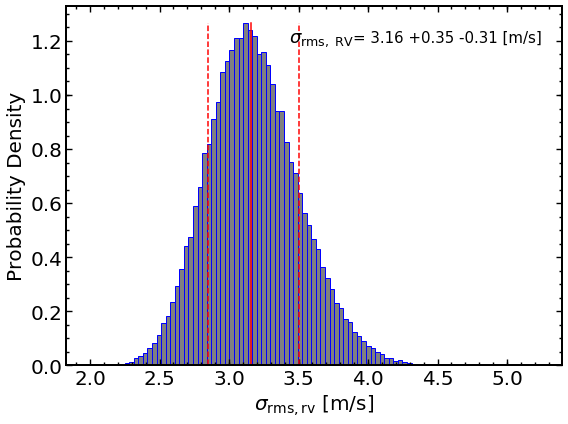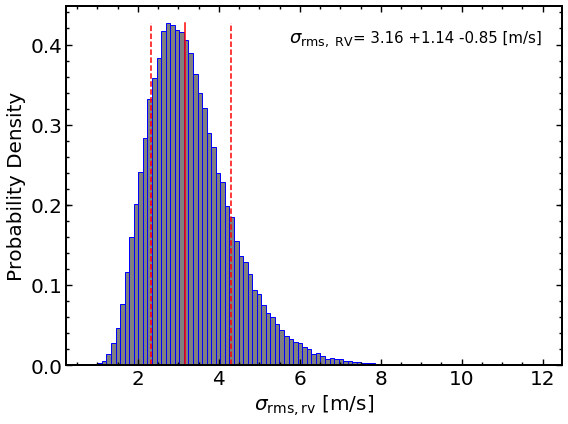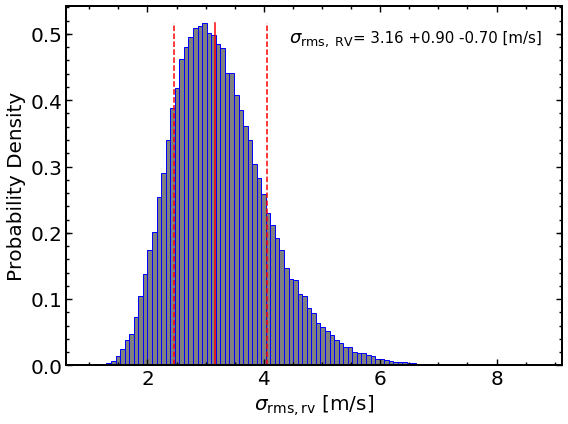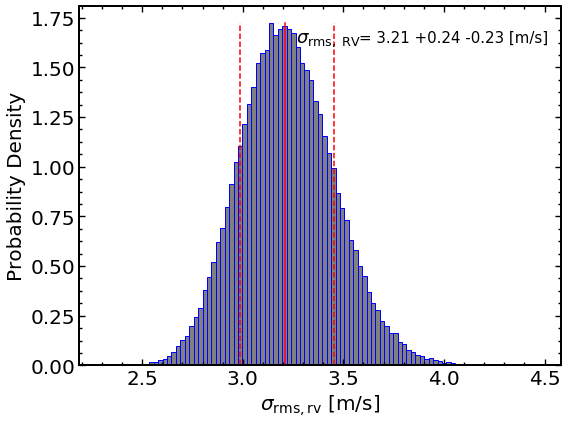In :
# Predict RV jitter median +/- one sigma, and MC simulation. Note that
# the RV jitter is firstly predicted with sole contribution from stellar
# oscillations, and then multiplied by a recommended factor, which differs slightly in
# different models, to include an additional source, granulation. The correction
# factor can be reset via "CorFact", such as CorFact=2.0.
import RVJitter
target = RVJitter.rvjitter(lumi=12.006, lumierr=1.131, mass=1.304, masserr=0.064, teff=4963.00, tefferr=80.000)
sigmarv, sigmarvperr, sigmarvmerr, mcsigmarv = target.rv()


In :
# Visualize the Monte Carlo simulation.
# Predict RV jitter from luminosity, mass, and effectice temperature.
import RVJitter
target = RVJitter.rvjitter(lumi=12.006, lumierr=1.131, mass=1.304, masserr=0.064, teff=4963.00, tefferr=80.000)
sigmarv, sigmarvperr, sigmarvmerr, mcsigmarv = target.plot(figshow=True, figsave=True, figname='jitter.png')In :
# Predict RV jitter from luminosity, effectice temperature, and surface gravity.
import RVJitter
target = RVJitter.rvjitter(lumi=12.006, lumierr=1.131, teff=4963.00, tefferr=80.000, logg=3.210, loggerr=0.006)
sigmarv, sigmarvperr, sigmarvmerr, mcsigmarv = target.plot(figshow=True, figsave=True, figname='jitter.png')In :
import RVJitter
target = RVJitter.rvjitter(teff=4963.00, tefferr=80.000, logg=3.210, loggerr=0.006)
sigmarv, sigmarvperr, sigmarvmerr, mcsigmarv = target.plot(figshow=True, figsave=True, figname='jitter.png')In :
# Predict RV jitter from luminosity and effective temperature, in which case
# the evolutionary stage need to be specified, either giant (Lgiant=True) or
# dwarf/subgiant (False). A default dividing point, log(g)=3.5, is adopted.
import RVJitter
target = RVJitter.rvjitter(lumi=12.006, lumierr=1.131, teff=4963.00, tefferr=80.000, Lgiant=False)
sigmarv, sigmarvperr, sigmarvmerr, mcsigmarv = target.plot(figshow=True, figsave=True, figname='jitter.png')In [ ]: